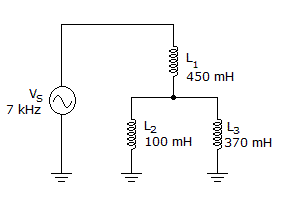# Electronics - Inductors

### Exercise :: Inductors - Filling the Blanks

1.

After a voltage has been applied to an inductor, the current will be ___ of its full value after 2 time constants.

 A. 63% B. 86% C. 95% D. 98%

Answer: Option B

Explanation:

No answer description available for this question. Let us discuss.

2.

The ___ of a coil is the inherent resistance of the wire.

 A. ac resistance B. reactance C. winding resistance D. permeability

Answer: Option C

Explanation:

No answer description available for this question. Let us discuss.

3.The inductive reactance of L2 is _____ in the given circuit.

 A. 3.5 kB. 4.4 kC. 16.3 kD. 23.3 kAnswer: Option B

Explanation:

No answer description available for this question. Let us discuss.

4.

___ power represents the energy dissipated at a given point in time.

 A. True B. Reactive C. Real D. Instantaneous

Answer: Option D

Explanation:

No answer description available for this question. Let us discuss.

5.

After the first time constant, a transient current has changed by _______________ %, leaving _______________ % remaining.

 A. 63.2, 36.8 B. 36.8, 63.2 C. 13.5, 86.5 D. 86.5, 13.5

Answer: Option A

Explanation:

No answer description available for this question. Let us discuss.

#### Current Affairs 2021

Interview Questions and Answers